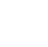Back to Chapter
Java IntroductionJava BasicsBranching and LoopingClasses, Objects, and MethodsArrays, Strings, and VectorsPackagesErrors and ExceptionApplet ProgrammingGraphics ProgrammingManaging I/O FilesJava Database Connectivity# Java Dynamic Arrays

The dynamic array is a variable size list data structure. It grows automatically when we try to insert an element if there is no more space left for the new element. It allows us to add and remove elements. It allocates memory at run time using the heap. It can change its size during run time. Dynamic arrays are two types:

# 1.Vectors

The Vector class is used to create a generic dynamic array known as vectors that can hold objects of any type and any number. It is contained in java.util package. Arrays can be easily implemented as vectors.

## Creating vectors:

Vectors are created like arrays as follows:

Vector <type> vector_name = new Vector <type> (); //declaring without size
Vector <type> vector_name = new Vector <type> (3);// declaring with size

1. It is convenient to use vectors to store objects.
2. A vector can be used to store a list of objects that may vary in size.
3. We can add and delete objects from the list.

Example 1: string type

import java.util.*;
public class VectorExample {
public static void main(String args[]) {
//Create a vector
Vector < String > vec = new Vector < String > ();

System.out.println("Elements of vector: " + vec);
}
}
/*OUTPUT:
Elements of vector: [Apple, Banana, Orange, Mango, Grapes, Watermelon, Gauva]

Example 2: integer type

import java.util.*;
public class VectorExample {
public static void main(String args[]) {
//Create an empty Vector
Vector < Integer > num = new Vector <> ();

//Display the vector elements
System.out.println("Values in vector: " + num);
//use remove() method to delete the first occurence of an element
System.out.println("Remove first occourence of 20:" + num.remove((Integer)20));
//Display the vector elements afre remove() method
System.out.println("Values in vector: " + num);;

}
}
/*OUTPUT:
Values in vector: [10, 20, 30, 20, 40]
Remove first occourence 20: true
Values in vector: [10, 30, 20, 40] */

# 2. ArrayList

An ArrayList class is a dynamic array, which is present in the java. util package. While built-in arrays have a fixed size, ArrayListcan change their size dynamically. Elements can be added and removed from an ArrayList whenever there is a need, helping the user with memory management.

The important points about Java Array List class are:

• Java Array List class can contain duplicate elements.
• Java Array List class maintains insertion order.
• Java Array List allows random access because array works at the index basis.

## Creating ArrayList:

Array List is created like arrays as follows:

syntax:

ArrayList<Type> arrayList= new ArrayList<>();

import java.util.ArrayList;

class Main {
public static void main(String[] args){

// create ArrayList
ArrayList<String> fruits = new ArrayList<>(); // ArrayList with String type

System.out.println("ArrayList: " + fruits);
}
}

/*OUTPUT:
ArrayList: [apple, mango, banana] */